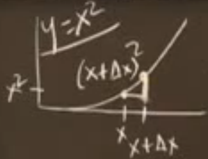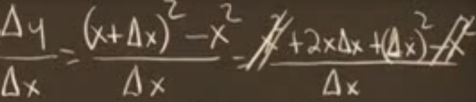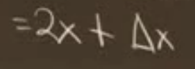# 微积分

Posted by allon li on 2017-11-22

# 微积分

### 微分 - 从函数一求函数二就是微分

△y、△x表示很小的一段，dy、dx表示极限小接近0但不是0。

$\frac{dy}{dx} = lim\frac{\Delta{y}}{\Delta{x}}$• $\frac{dy}{dx}$ 简写为 y`，二阶导数简写为y’’
• 一阶导数正负表示增长，二阶导数正负表示凹凸。凹：向下弯曲；凸：向上弯曲。
• 拐点的 y’’=0

### 积分 - 从函数二反求函数一就是积分（原函数是其导数的积分）

• 方法一：函数一是函数二倒过来的函数，分别有算术法和代数（字母）法。通过推导哪些函数的微分函数是已知函数。【主要是求和】
• 方法二：函数一是函数二图像下的面积。通过面积求原函数，原函数是x到0围成的面积。

### 乘法和除法法则

$P(x) = f(x).g(x)$

$\frac{dP}{dx}=f(x)\frac{dg}{dx} + g(x)\frac{df}{dx}$

$f(x) = x^n$

f(x)的导数为：

$\frac{df(x)}{dx}=\frac{d}{dx}x^n=nx^{n-1}$

$\frac{d}{dx}(\frac{f(x)}{g(x)})=\frac{\frac{g(x).df(x)}{dx}-\frac{f(x).dg(x)}{f(x)}}{g(x)^2}$

### 链式法则

$\frac{dz}{dx}=\frac{dz}{dy}\frac{dy}{dx}$